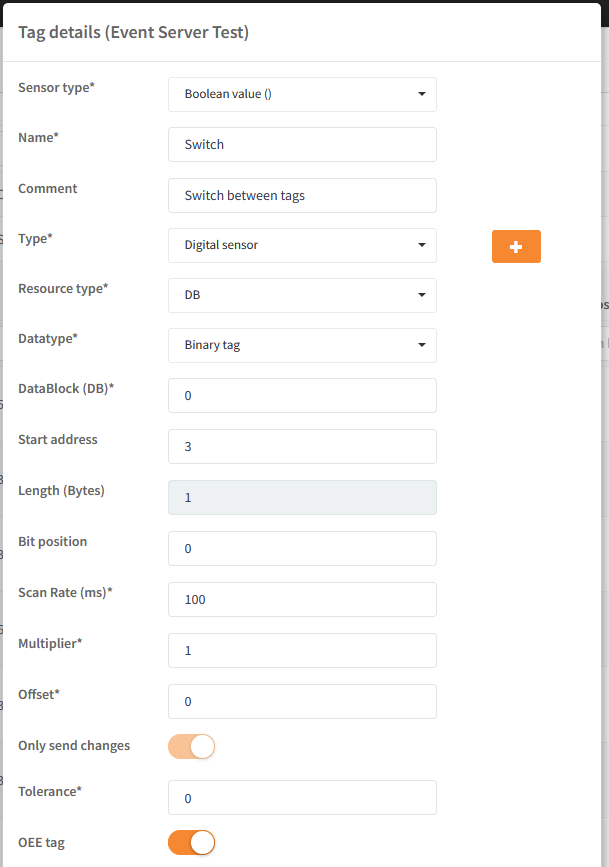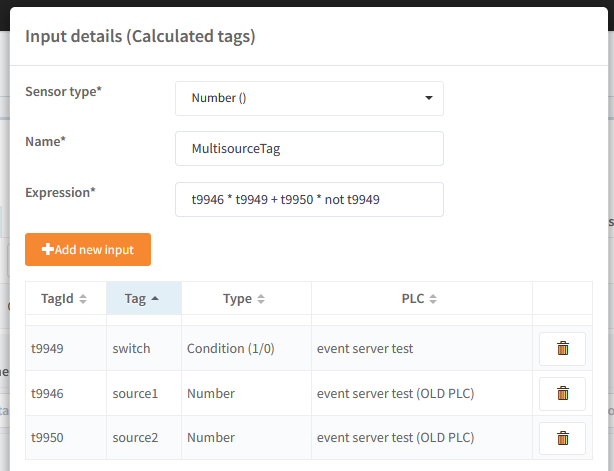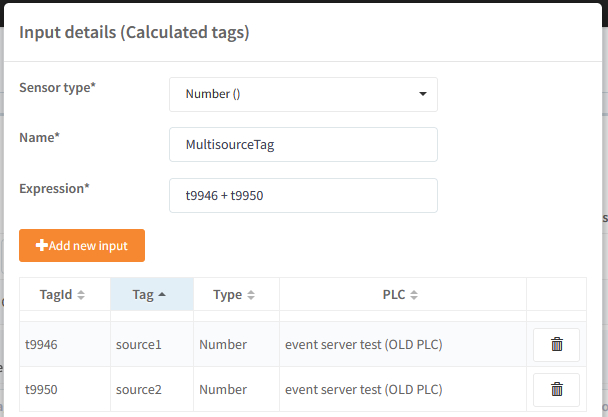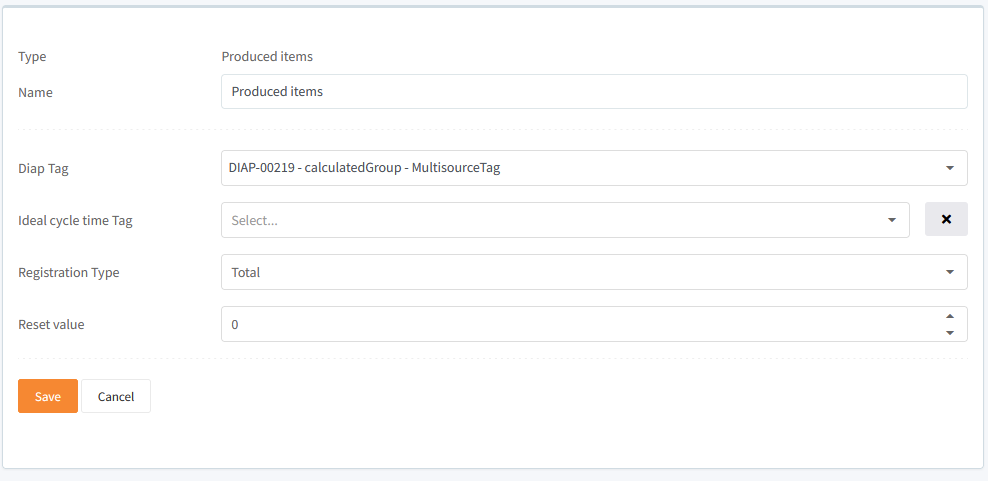# Example: Calculated tags for selecting tag source

This guide assumes, that you know about calculated inputs, if you don't you can read more about them here: calculated inputs

### Creating a multi source tag

You can use calculated input as selector for which tag data should be used.  This calculated input will switch between tags. This can be achieved with 3 tags:

 Tag name data type Description Switch bit/int/float (a number) The switch, which decide which source will be used Source1 bit/int/float (a number) First data source Source2 bit/int/float (a number) Second data source

The tag setting for the switch, could look like this:and a tag setting for one of the source tags, could look like this:With these tags, you can make an expression in a calculated input, which will switch between 2 tags:

`Source1 * Switch + Source2 * not SwitchExample:Switch = 0Source1 = 7Source2 = 27 * 0 + 2 * not 0 = 0 + 2 * 1 = 2Switch = 1 7 * 1 + 2 * not 1 = 7 + 2 * 0 = 7`

The calculated input setting for this expression could look like this:### Creating a multi source tag with only 2 tags

You can make the multi source tag, which only uses 2 tags:

 Tag name data type Description Source1 bit/int/float (a number) First data source, is 0 when not active Source2 bit/int/float (a number) Second data source, is 0 when not active

The big difference between this setup and the one with the switch tag, is that when a tag shouldn't be used, it is set to 0. With this setup, you make the following expression in a calculated input, which will switch between 2 tags:

`Source1 + Source2Example:Source1 = 7Source2 = 0 (not active)7 + 0 = 7Source1 = 0 (not active)Source2 = 2 0 + 2 = 2`

The calculated input setting for this expression could look like this:### Use multi source tag in OEE.

You can these multi source tag as the data source for the production on a OEE unit:(you can read more about OEE production here Setup registration of production counters)